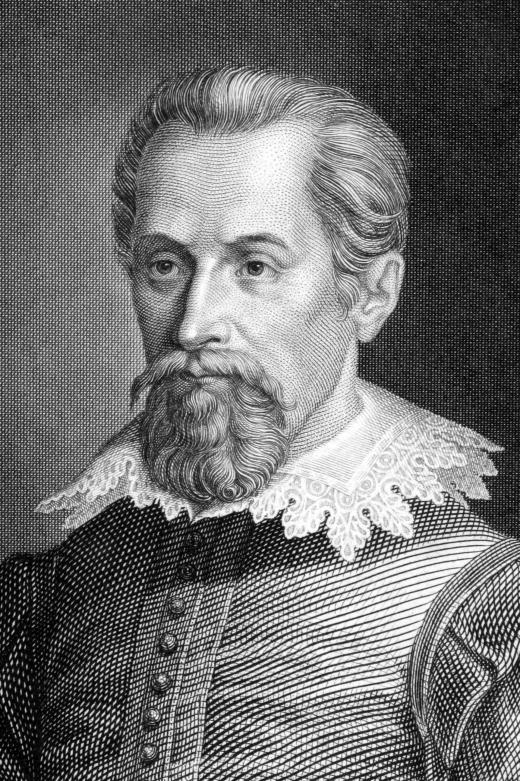Science
Fact Checked

# What Is Angular Momentum?

Michael Smathers
Michael Smathers

Momentum is a measurement of motion that determines how much force an object of a given mass will exert when traveling at a set velocity. The equation for straight-line momentum is simple: p = mv, where p is momentum and m and v are mass and velocity. Angular momentum is a slightly different quantity; it involves calculating the motion of an object or particle around a fixed point, a system also known as an orbit. Calculating angular momentum differs slightly for particles and objects, but is similar to calculating linear momentum.

The formula for angular momentum of particles is L = rp. L is the momentum, r is the radius from the center of the orbit to the particle and p is the linear momentum of the particle: mass times velocity. Angular momentum as it applies to objects is somewhat different; the formula is L = Iω, where L is the momentum, I is the moment of inertia, and ω is the angular velocity. An important concept, the moment of inertia affects torque, or rotational force around a fixed axis. The moment of inertia is the product of the mass and the square of the rotational radius, or I = mr2.Astronomer Johannes Kepler used angular momentum to develop one of his planetary motion laws.

An object's momentum around its axis causes the axis to remain stationary — regardless of the weight attached to it — when the mass is moving quickly, similar to the motion of a spinning top. In other words, the rotational motion of a rapidly-spinning body causes the axis to stabilize. For example, a bicyclist has an easier time staying upright when the bike's wheels are spinning quickly. Similarly, football players give the ball a spiraling motion to make it fly straighter toward their teammate, and by the same principle, a gun barrel includes rifling along the interior of the barrel to give the bullet a spiral rotation as it travels.

Calculating angular momentum is useful in determining the orbits of celestial bodies. Johannes Kepler, a 17th-century Dutch astronomer, developed his Second Law of Planetary Motion via the concept of conservation of angular momentum. This law states that as long as there is no external torque on an object in orbit, its momentum will never change. As it gets closer to the center of rotation, its rotational speed will increase, and it will decrease the farther away it gets from the axis of rotation.

## You might also Like